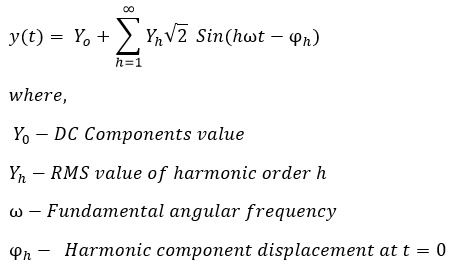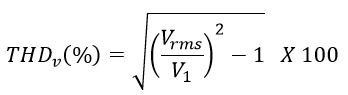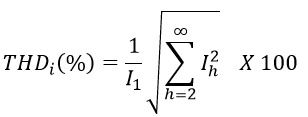Search

# Total Harmonic Distortion & Its Measurement

If the sinusoidal voltage is applied to linear devices like inductor,capacitor and resistor, the current drawn by these devices varies according to the variation of sinusoidal voltage. In this case, both voltage and current waveform do not introduce any harmonics in the supply network.

If the sinusoidal voltage applied to non linear semiconductor devices, the current flowing through these devices are not vary as per the applied sinusoidal voltage. The distorted current waveform introduce harmonics in the system. The current distortion further exacerbate if the applied voltage is also distorted.The distortion in voltage and current waveform caused by harmonics is called harmonic distortion. Thus, the harmonics pollute the supply system and decrease the efficiency of electrical supply network.

According to Fourier series,the distorted non-sinusoidal waveform can be resolved into following components.

1. Fundamental frequency as a sinusoidal component

2. Integral multiples of fundamental frequency components

3. DC componentsIf the fundamental frequency is 50 C/s. The harmonic frequency is 100,150,200,250,300.... Hz etc. The even multiples of fundamental frequency 100,200,300.... Hz etc is known as even harmonics and , the odd multiples of fundamental frequency 150,250,350.... Hz etc is known as odd harmonics.The distorted waveform symmetrical about x-axis contains only odd harmonics.

The harmonics cause numerous problems in the supply network and also pernicious for electrical equipment health, therefore measurement of harmonics and its mitigation is must for maintaining the power system efficiency.

The total harmonic distortion is measured to determine the degree of harmonics present in the power supply system. For a signal m, the total harmonic distortion is defined by following equation.The total harmonics distortion of current and voltage is measured in terms of THDv and THDi. The harmonic current drawn by no linear devices cause voltage distortion and again the distorted voltage cause current distortion. Therefore, it is very important to mitigate the harmonic current to avoid electrical pollution.

## Current and Voltage Total Harmonic Distortion(THD)

The total harmonic distortion of voltage (THDv) is defined as the ratio of sum of root means squares of all the harmonic voltages to the RMS voltage of fundamental frequency. The equation of total harmonic distortion of voltage in percentage is as given below.The generalized equation of total harmonic distortion of voltage is;If the total RMS value of distorted voltage waveform is known, the total harmonic distortion of voltage can be calculated by following equation.The total harmonic distortion of current (THDi) is defined as the ratio of sum of root means squares of all the harmonic currents to the RMS current of fundamental frequency. The equation of total harmonic distortion of current in percentage is as given below.The generalized equation of total harmonic distortion of current is;If the total RMS value of distorted current waveform is known, the total harmonic distortion of current can be calculated by following equation.